# Area of Plane Shapes

The area of a flat shape is the amount of space it takes up. In other words, it is the size of a surface.

Let's start with the formulas for the area of some plane shapes, and then look at a few examples.

## Area Formulas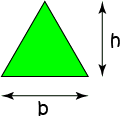Triangle

$\text{Area} = \dfrac{1}{2} \times b \times h$

$b$ is the length of the base, and $h$ is the height of the triangle.Square

$\text{Area} = s^2$

$s$ is the side-length of the square.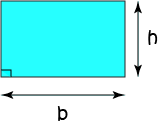Rectangle

$\text{Area} = b \times h$

$b$ is the base (or length) of the rectangle.

$h$ is the height of the rectangle.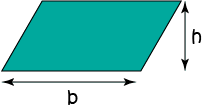Parallelogram

$\text{Area} = b \times h$

$b$ is the base (or length) of the parallelogram.

$h$ is the height of the parallelogram.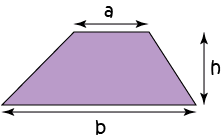Trapezium

$\text{Area} = \dfrac{1}{2}(a + b) \times h$

$a$ and $b$ are the parallel sides of the trapezium.

$h$ is the height of the trapezium.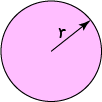Circle

$\text{Area} = \pi r^2$

$r$ is the radius of the circle.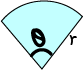Sector

$\text{Area} = \dfrac{1}{2} \times r^2 \times \theta$

Note: for this to work, the angle must be measured in radians

Here, $r$ is the radius and $\theta$ is the angle at the centre, in radians.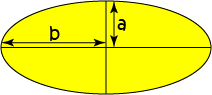Ellipse

$\text{Area} = \pi ab$

$a$ and $b$ are half the lengths of the axes of the ellipse.

Note: In the above, the height is always perpendicular to the base of the shape.

## Example 1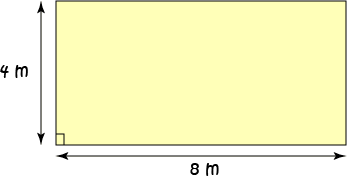What is the area of this rectangle?

Solution: The base has length $8$ m, and the height is $4$ m, so the area is

$8 \times 4 = 32 \text{ m}^2$

## Example 2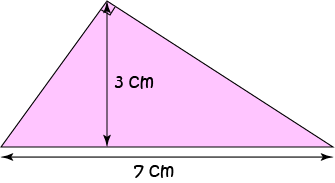What is the area of this triangle?

Solution: The base has length $7$ cm, and the height is $3$ cm, so the area is

$\dfrac{1}{2} \times 7 \times 3 = 10.5 \text{ cm}^2.$

## Example 3What is the area of this trapezium?

Solution: The parallel sides have lengths $4$ m and $7$ m, and the height is $2$ m, so the area is

$\dfrac{1}{2} \times (4 +7) \times 2 = 11 \text{ m}^2.$

## One Last Example

Sam has a part-time job, mowing the oval-shaped park shown below.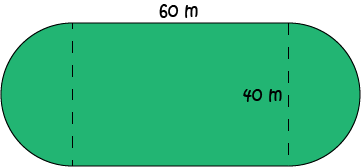If he is paid $\0.02$ per square metre mown, how much does he earn each time he mows the park?

Solution: We need to find the area of the oval in square metres and multiply it by $\0.02$ to find Sam's earnings.

The oval is made up of two straight sides of length $60$ m, and two semi-circles of radius $\dfrac{1}{2}(40) = 20 \text{ m}$. So, its area is equal to the area of a circle of radius $20$ m, plus the area of a rectangle with base length $60$ m and height $40$ m:

\begin{align*} \text{Area} &= \pi r^2 + b \times h\\ &= \pi (20)^2 + (60)(40)\\ &= 400 \pi + 2,400 \text{ m}^2\\ &\approx 3656.64 \text{ m}^2. \end{align*}

So, Sam is paid

$\text{Wage} = 3656.64 \times 0.02 = \73.13$
for the job.

### Description

In these chapters you will learn about plane geometry topics such as

• Area (Irregular polygons, plane shapes etc)
• Perimeter
• Conic sections (Circle, Ellipse, Hyperbola etc)
• Polygons (Congruent, polygons, similar, triangles etc)
• Transformations and symmetry (Reflection, symmetry, transformations etc)

etc

Even though these chapters are marked for Year 10 or higher students, several topics are for students in Year 8 or higher

### Audience

Year 10 or higher, suitable for Year 8 + students as well.

### Learning Objectives

You must be logged in as Student to ask a Question.